Successfully reported this slideshow.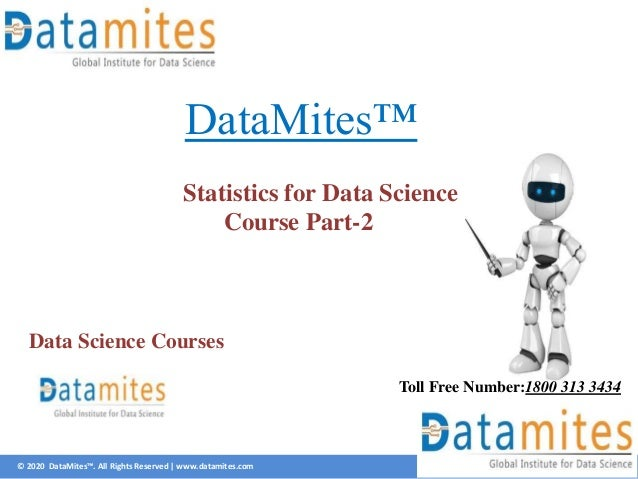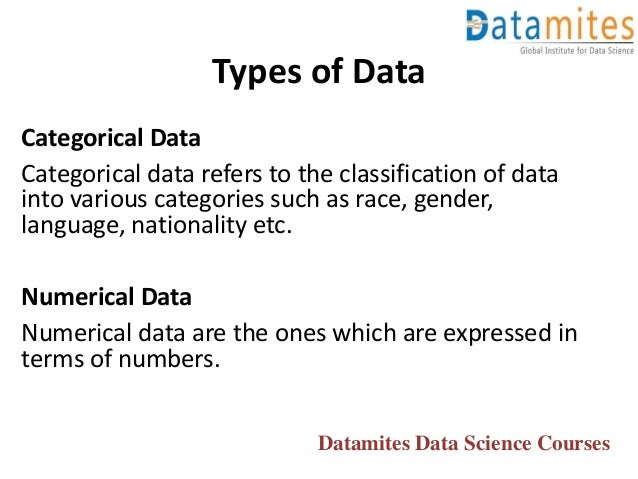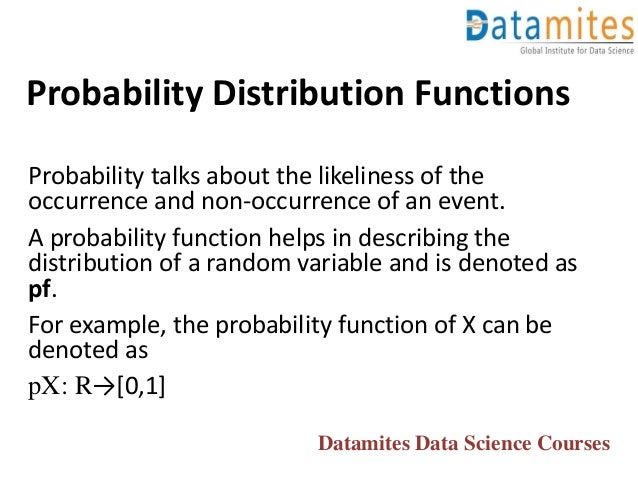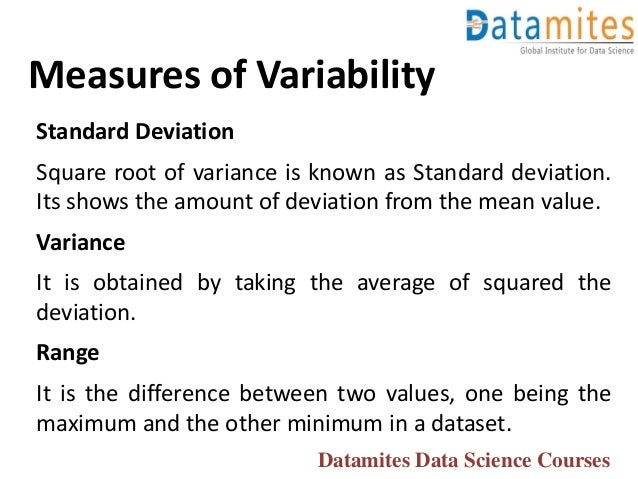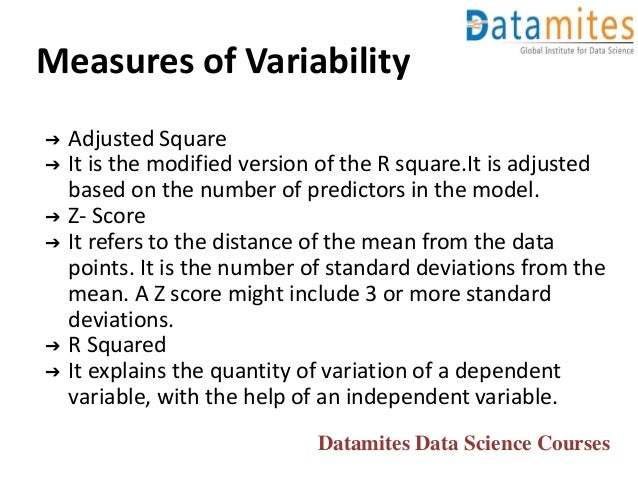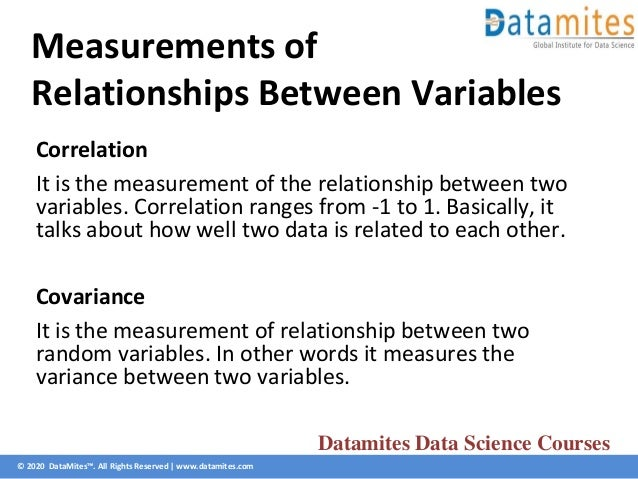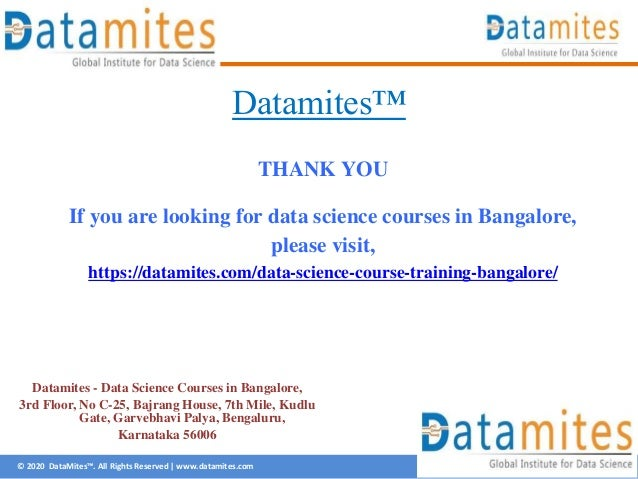Upcoming SlideShare
×

# Statistics For Data Science Course Part 2

Data Scientist and Analyst Can look for meaningful changes in data and Statistics can be used to derive meaningful insights from Data.

• Full Name
Comment goes here.

Are you sure you want to Yes No• Be the first to comment

• Be the first to like this

### Statistics For Data Science Course Part 2

1. 1. © 2020 DataMites™. All Rights Reserved | www.datamites.com DataMites™ Statistics for Data Science Course Part-2 Toll Free Number:1800 313 3434 Data Science Courses
2. 2. Categorical Data Categorical data refers to the classification of data into various categories such as race, gender, language, nationality etc. Numerical Data Numerical data are the ones which are expressed in terms of numbers. Types of Data Datamites Data Science Courses
3. 3. Probability Distribution Functions Probability talks about the likeliness of the occurrence and non-occurrence of an event. A probability function helps in describing the distribution of a random variable and is denoted as pf. For example, the probability function of X can be denoted as pX: R→[0,1] Datamites Data Science Courses
4. 4. Measures of Variability Standard Deviation Square root of variance is known as Standard deviation. Its shows the amount of deviation from the mean value. Variance It is obtained by taking the average of squared the deviation. Range It is the difference between two values, one being the maximum and the other minimum in a dataset. Datamites Data Science Courses
5. 5. Measures of Variability ➔ Adjusted Square ➔ It is the modified version of the R square.It is adjusted based on the number of predictors in the model. ➔ Z- Score ➔ It refers to the distance of the mean from the data points. It is the number of standard deviations from the mean. A Z score might include 3 or more standard deviations. ➔ R Squared ➔ It explains the quantity of variation of a dependent variable, with the help of an independent variable. Datamites Data Science Courses
6. 6. © 2020 DataMites™. All Rights Reserved | www.datamites.com Measurements of Relationships Between Variables Correlation It is the measurement of the relationship between two variables. Correlation ranges from -1 to 1. Basically, it talks about how well two data is related to each other. Covariance It is the measurement of relationship between two random variables. In other words it measures the variance between two variables. Datamites Data Science Courses
7. 7. © 2020 DataMites™. All Rights Reserved | www.datamites.com Datamites™ THANK YOU If you are looking for data science courses in Bangalore, please visit, https://datamites.com/data-science-course-training-bangalore/ Datamites - Data Science Courses in Bangalore, 3rd Floor, No C-25, Bajrang House, 7th Mile, Kudlu Gate, Garvebhavi Palya, Bengaluru, Karnataka 56006

Total views

11

On Slideshare

0

From embeds

0

Number of embeds

0

1

Shares

0